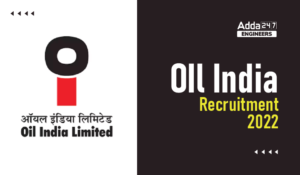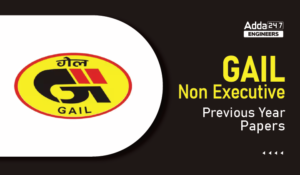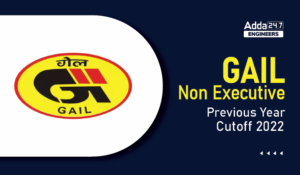Engineering Jobs   »   QUIZ : CIVIL ENGINEERING (13-04-2021)

# QUIZ : CIVIL ENGINEERING (13-04-2021)

Quiz: Civil Engineering

Exam: PUNJAB PSC-JE

Topic: Miscellaneous

Each question carries 2.5 mark

Negative marking: NO NEGATIVE MARKING

Time: 10 Minutes

Q1. If the depth is 8.64 cm on a field over a base period of 10 day, then the duty is

(a) 10 hectares per cumecs

(b) 100 hectares per cumecs

(c) 864 hectares per cumecs

(d) 1000 hectares per cumecs

Q2. Bending moment M and torque T is applied on a solid circular shaft. if the maximum bending stress equals to maximum shear stress developed, the M is equals to

(a) T/2

(b) T

(c) 2T

(d) 4T

Q3. If the D10, D30, D60 sizes of the soil mass is found to be 0.15 mm, 0.35mm, and 0.75 mm respectively, then the coefficient of curvature of the soil mass from line analysis of particle size distribution curve will be

(a) 2.14

(b) 5.0

(c) 1.08

(d) 10.8

Q4. As per IS code, the minimum grade of concrete for the design of prestressed concrete structure is:

(a) M20

(b) M25

(c) M15

(d) M30

Q5. In a slab, the minimum reinforcement using steel of grade Fe 415 is:

(a) 0.12% of its gross sectional area

(b) 0.20% of its gross sectional area

(c) 0.15% of its gross sectional area

(d) 0.10% of its gross sectional area

Q6. Span to effective depth ratio for a continuous beam with steel grade of Fe 415 should not be more than:

(a) 7

(b) 20

(c) 26

(d) 48

Q7. The maximum depth of neutral axis in a RC beam for Fe 415 grade of steel is:

(a) 0.53d

(b) 0.46d

(c) 0.67d

(d) 0.48d

Q8. For a statically indeterminate pin jointed plane frame, the relation between number of member ‘m’ and number of joints ‘j’ is expressed as:

(a) m=3j-6

(b) m>2j-3

(c) m=2j-3

(d) m>3j-6

Q9. Bulk modulus is defined as ratio of:

(a) Direct stress and volumetric strain

(b) Longitudinal stress and shear strain

(c) Shear stress and shear strain

(d) Volumetric stress and volumetric strain

Q10. Which of the following sections will have large shape factor?

(a) Triangle

(b) Rectangle

(c) I-section

(d) Solid circular section

SOLUTION

S1. Ans. (d)
Sol. Given, ∆ =8.64 cm = 0.0864 m, B = 10 days
Duty (D) = (8.64 × B)/(∆ ) = (8.64 × 10)/(0.0864 ) = 1000 hectares per cumecs.

S2. Ans. (a)
Sol. Maximum Bending Stress (σ_max)=32M/(πD^3 )
Maximum Shear Stress (τ_max)=16T/(πD^3 )
σ_max= τ_max (as per question)

32M/(πD^3 )= 16T/(πD^3 )

M = T/2

S3. Ans. (c)
Sol. Coefficient of Curvature (CC)= (〖D_30〗^2 )/(D_60×D_10 )=〖0.35〗^2/(0.75×0.15)=1.08

S4. Ans.(d)

Sol. As per IS 456:2000, The Minimum M30 grade of concrete is used in post tensioned member and M40 grade of concrete is used in pre tensioned member.

S5. Ans.(a)

Sol. In a slab, the minimum reinforcement using steel of grade HYSD bars (Fe 415) is 0.12% of its gross sectional area and for plain bars (Fe 250), the minimum reinforcement is 0.15% of its gross sectional area.

S6. Ans.(c)

Sol. As per IS code–

S7. Ans.(d)

Sol.

Where, d= effective depth

S8. Ans.(b)

Sol. m + 3 = 2jStatic Determinate structure

m + 3 > 2jStatic Indeterminate structure

m + 3 < 2jUnstable structure

S9. Ans.(a)
Sol. Bulk modulus (K) = (Direct Stress)/(Volumetric strain)

S10. Ans.(a)

Sol.

Sharing is caring!

Thank You, Your details have been submitted we will get back to you.
•DVC Interview Schedule 2022, Download DV...
•ONGC Non Executive Answer Key 2022, Down...
•Oil India Recruitment 2022, Apply Online...
•GAIL Non Executive Previous Year Papers,...
•GAIL Non Executive Previous Year Cutoff ...
•APWD Previous Year Papers, Check here fo...